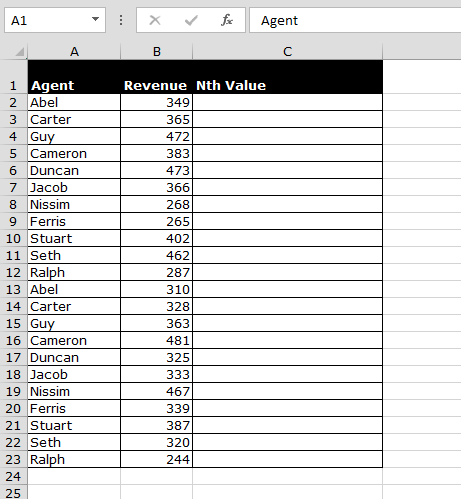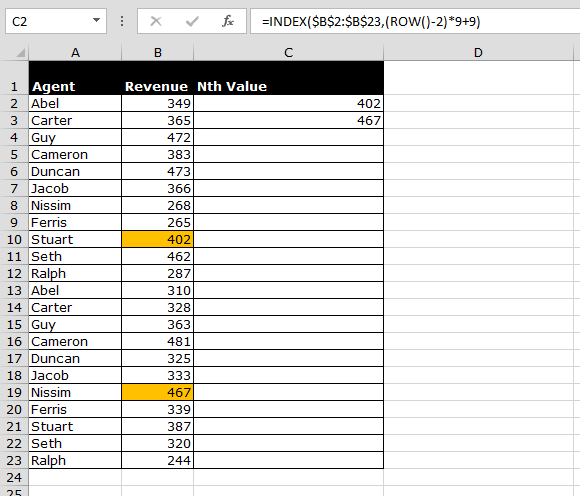# How to Retrieve Every Nth Value in a Range in Excel

In this article, we will learn how to retrieve every nth value in a range. We will use Index function with Row function.

Why and how we use Index and row function?

We use Index function to return value from the intersection between the row number and the column number in an Array. This function will pick the number and give use as output.

Row function is used to return the row number of a cell reference. So in this situation row function will help to pick every nth value from range.

Let’s take an example and understand how we use Index and row function?

Let’s take an example to understand how we can retrieve the Nth value in a row, column and range.

We have Agent data in Excel. Column A contains agent name and column B contains revenue. From column B, we want to retrieve every nth value.Follow below steps to retrieve the Nth value from the list:-

• Enter the formula in cell C2.
• =INDEX(\$B\$2:\$B\$23,(ROW()-2)*9+9)
• Press Enter.
• Copy the same formula in next cells according to data.
• Below you can see formula has returned every nth value in column C.Note: - If you want to return every 3rd value, then you just need to change in formula 3 at the place of 9, and formula will pick every 3rd value from the range.

This is the way we can use Excel formulas Index and row to get the Nth, 3rd, 4thetc. value from the range.We would love to hear from you, do let us know how we can improve, complement or innovate our work and make it better for you. Write us at info@exceltip.com

Popular Articles :

50 Excel Shortcut to Increase Your Productivity : Get faster at your task. These 50 shortcuts will make you work even faster on Excel.

How to use the VLOOKUP Function in Excel : This is one of the most used and popular functions of excel that is used to lookup value from different ranges and sheets.

How to use the COUNTIF function in Excel : Count values with conditions using this amazing function. You don't need to filter your data to count specific values. Countif function is essential to prepare your dashboard.

How to use the SUMIF Function in Excel : This is another dashboard essential function. This helps you sum up values on specific conditions.

1.Donald Belfort

ANIS, you should be able to retrieve to another sheet by applying the same rules, but highlight the dataset you are attempting to extract the data from and hop back to the original sheet.

2.IF I WANT TO RETRIEVE TO ANOTHER SHEET ?

3.Can you tell me how to display every nth row to a single cell. That means every time the nth row is filled with data the last entered nth value should display in cell C2

4.Gamuchirai Moyo

I would want to create list 2 consisting of ever 10th value in a row, the formula given is not telling me every detail,

=INDEX(\$A\$2:\$A\$13,(ROW()-2)*3+3)
My confusion is on how to apply the 2, the 3s and empty brackets following the row, what should I fill in there, or they are supposed to be empty???